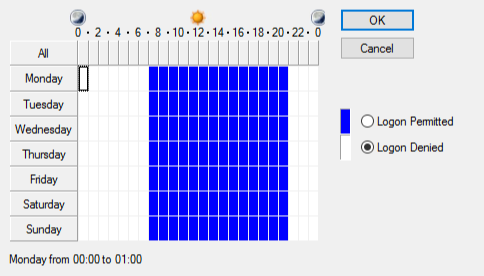# Log On hours with powershell

We had a requirement recently to set logon hours for a specific set of users. To achieve this, we set up one user through the AD User and computers snap-in:We then created a script (borrowing from other snippets/scripts we found on the web) that would interrogate the source users logon hours, and then allow us to apply them to all users in a group:
``````#############################################################
#         Simple script to get and set logon hours          #
#                                                           #
# Set up an account through ADUC and configure log on hours #
#                                                           #
#    This script will then enumerate this and set hours     #
#                                                           #
#                 on the group you specify                  #
#############################################################
\$validuser = \$false
\$validgroup = \$false

while(!(\$validuser)){
clear-host
write-host "
__                 ___
/ /  ___   __ _    /___\_ __     /\  /\___  _   _ _ __ ___
/ /  / _ \ / _  |  //  // '_ \   / /_/ / _ \| | | | '__/ __|
/ /__| (_) | (_| | / \_//| | | | / __  / (_) | |_| | |  \__ \
\____/\___/ \__, | \___/ |_| |_| \/ /_/ \___/ \__,_|_|  |___/
|___/
" -ForegroundColor Cyan
\$user = read-host Enter the user name of the log on hours you wish to duplicate:
If(\$null -ne \$testresult){
\$validuser = \$true
}Else{
Write-host "No such user!" -foregroundcolor red
start-sleep -seconds 2
}
}
write-Host Logon Hours for this user are: -ForegroundColor Green
\$logonhours = get-aduser \$user -property logonhours | select-object -expand logonhours
\$dn = (get-aduser \$user -property distinguishedname | select-object distinguishedname).distinguishedname
[byte[]]\$hours1 = \$logonhours

\$Bias = [Math]::Round((Get-ItemProperty `
-Path HKLM:\System\CurrentControlSet\Control\TimeZoneInformation).Bias/60, `
0, [MidpointRounding]::AwayFromZero)

# Create an empty array with 168 elements, one for each hour of the week.
\$LH = New-Object 'object[]' 168

Function OctetToHours (\$Octet)
{
# Function to convert Octet value (byte array) into binary string
# representing the logonHours attribute. The 168 bits represent 24 hours
# per day for 7 days, Sunday through Saturday. The logonHours attribute
# in Active Directory is in UTC. This function converts into the local
# time zone. If the bit is "1", the user is allowed to logon during
# that hour. If the bit is "0", the user is not allowed to logon.

If (\$Octet -eq \$Null)
{
# If the logonHours attribute has no value, the user is allowed
# logon all hours.
For (\$j = 0; \$j -le 167; \$j = \$j + 1)
{
\$LH[\$j] = "1"
}
}
Else
{
# Loop through the 21 bytes in the array, each representing 8 hours.
For (\$j = 0; \$j -le 20; \$j = \$j + 1)
{
# Check each of the 8 bits in each byte.
For (\$k = 7; \$k -ge 0; \$k = \$k - 1)
{
# Adjust the index into an array of hours for the
# local time zone bias.
\$m = 8*\$j + \$k - \$Bias
# The index into the  array of hours ranges from 0 to 167.
If (\$m -lt 0) {\$m = \$m + 168}
# Check the bit of the byte and assign the corresponding
# element of the array.
If (\$Octet[\$j] -band [Math]::Pow(2, \$k)) {\$LH[\$m] = "1"}
Else {\$LH[\$m] = "0"}
}
}
}

"Day"
"of   ------- Hour of the Day -------"
"Week M-3 3-6 6-9 9-N N-3 3-6 6-9 9-M"
\$arrDays = ("Sun", "Mon", "Tue", "Wed", "thu", "Fri", "Sat")

# Loop through the array of 168 hours.
For (\$j = 0; \$j -le 167; \$j = \$j + 1)
{
If ((\$j % 24) -eq 0)
{
# First hour of a day.
\$Line = \$arrDays[\$j / 24] + " "
}
If ((\$j %3) -eq 0)
{
# Insert a space every 3 hours for legibility.
\$Line = \$Line + " "
}
\$Line = \$Line + \$LH[\$j]
# If this is the last hour of the day, output.
If (((\$j + 1) % 24) -eq 0) {\$Line}
}
}

"User: " + \$User

OctetToHours \$mUser.logonHours
\$check = Read-host "Is this correct? (y/n)"
if(\$check -eq "n"){
write-host Please reconfigure the source account correctly and try again -ForegroundColor Red
Exit
}

while(!(\$validgroup)){
clear-host
write-host "
__                 ___
/ /  ___   __ _    /___\_ __     /\  /\___  _   _ _ __ ___
/ /  / _ \ / _  |  //  // '_ \   / /_/ / _ \| | | | '__/ __|
/ /__| (_) | (_| | / \_//| | | | / __  / (_) | |_| | |  \__ \
\____/\___/ \__, | \___/ |_| |_| \/ /_/ \___/ \__,_|_|  |___/
|___/
" -ForegroundColor Cyan
write-host Source Account is \$user -ForegroundColor Green
\$group = read-host Enter the name of the group you wish to apply this to:

\$GroupExists = Get-ADGroup -LDAPFilter "(SAMAccountName=\$group)" -ErrorAction SilentlyContinue
If(!\$GroupExists){
Write-host "No such group!" -foregroundcolor red
start-sleep -seconds 2
}Else{
write-host Group Exists!
\$validgroup = \$true
}
}
#ok we have a valid group, lets proceed...
\$members = Get-ADGroupMember -Identity \$group -Recursive | Select -ExpandProperty samAccountName

# Iterate through each member of the current group
ForEach (\$member in \$members) {